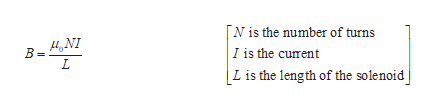# Consider a solenoid of length L, N windings, and radius b (L is much longer than b). A current I is flowing through the wire. If the radius of the solenoid were doubled (becoming 2b), and all other quantities remained the same, the magnetic field is same, half, twice as strong?  Need to understand the concept.

Question
26 views

Consider a solenoid of length L, N windings, and radius b (L is much longer than b). A current I is flowing through the wire. If the radius of the solenoid were doubled (becoming 2b), and all other quantities remained the same, the magnetic field is same, half, twice as strong?  Need to understand the concept.

check_circle

Step 1

Let the length of the solenoid be L, number of windings be N, and radius be b.

Step 2

Write the expression for the magneti...help_outlineImage Transcriptionclose[N is the number of turns I is the curent Lis the length of the solenoid B 4NI L fullscreen

### Want to see the full answer?

See Solution

#### Want to see this answer and more?

Solutions are written by subject experts who are available 24/7. Questions are typically answered within 1 hour.*

See Solution
*Response times may vary by subject and question.
Tagged in

### Magnetic Effects of Current